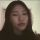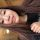# Real models of spherical homogeneous spaces

Лекция
Предмет:
Курс лекций:
Дата записи:
23.08.18
Дата публикации:
14.09.18
Код для блога:

Let G be a connected reductive algebraic group over the field of complex numbers C. Let Y=G/H be a spherical homogeneous space of G (a homogeneous space of special kind). Let G_0 be a real model (real form) of G, that is, a model of G over the field of real numbers R.
In the talk I will discuss the following question: does there exist a G_0 -equivariant real model Y_0 of Y? This is interesting even in the case when G=G'xG', where G' is a connected semisimple group over C, and H=G' embedded diagonally into G'xG'.
No preliminary knowledge of sperical varieties will be assumed.

7

## КомментарииСпасибо за очень познавательное видео!Очень интересно!Посмотрела... познавательная лекция!